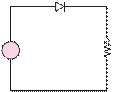### Create an Account

Home / Questions / The diode in the circuit shown in Figure P927 is fabricated from silicon and

# The diode in the circuit shown in Figure P927 is fabricated from silicon and

The diode in the circuit shown in Figure P9.27 is fabricated from silicon and

iIo(evD/V− 1where at = 300 K

kT

9.34  In the rectifier circuit shown in Figure P9.34,

v(t) sin(2π 100)V. Assume a forward voltage drop of 0.7 V across the diode when it is conducting. If conduction must begin during each positive half-cycle at an angle no greater than 5◦, what is the minimum peak value that the AC source must produce?q

I= 250 × 10−12 A        VT  =

≈ 26 mV

vVv= 4.2V + 110 cost)     mV

ω = 377 rad/s        = 7 kQ

The DC operating point or quiescent point (point) and the AC small-signal equivalent resistance at this point are

IDQ  = 0.548 mA        VDQ  = 0.365 V       rd  = 47.45 Q

Determine, using superposition, the AC voltage across the diode and the AC current through it.

Jun 18 2020 View more View LessSubscribe To Get Solution